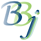# OEM Type

By default, numeric columns that end in the Date Column Suffix will be treated as Julians and converted to SQL_DATES.

If using an OEM database that has a non-Julian proprietary date format, then select one of the six corresponding OEM date types listed below to indicate the OEM date preference for character column names ending in the Data Column Suffix. This will override the default setting (registry keyword: datetype).

The OEM date type options are:

 Keyword Year Month Day AON asc(c\$(1,1))-32 + 1900num(c\$(1,4))num(c\$(1,2)) asc(c\$(2,1))-32num(c\$(5,2))num(c\$(3,2)) asc(c\$(3,1))-32num(c\$(7,2))num(c\$(5,2)) For the year, the third AON type works this way:For years 00-99, the value is 1900+the year. For example, 15 would be 1915. For years > 1999, a lettering system is used.A0 - A9 evaluate to 2000-2009.B0-B9 evaluate to 2010-2019.C0-C9 evaluate to 2020-2029, etc. CITI num(hta(c\$(1,1))) num(hta(c\$(2,1))) num(hta(c\$(3,1))) DISC (See below) MAI (Pre Y2K) asc(c\$(1,1))-129 + 1900 asc(c\$(2,1))-129 asc(c\$(3,1))-129 MAI (Post Y2K) asc(c\$(1,1))-32 + 1900 asc(c\$(2,1))-32 asc(c\$(3,1))-32 SOA asc(c\$(1,1))-32*64+asc(c\$(2,1))-32 num(c\$(3,2)) num(c\$(5,2)) SSI SSI num(c\$(1,2))num(c\$(1,4)) num(c\$(3,2))num(c\$(5,2)) num(c\$(5,2))num(c\$(7,2)) SSIJ HTA() of a 3 byte Julian Integer For SSIJ to receive a Julian value from the stored version of the SSIJ date format, use the following formula: julian = dec(ath(c\$)); To create a SSIJ date from a year, month, and day: s\$=hta(bin(jul(year,month,day),3)); YMD8 num(c\$(1,4)) num(c\$(5,2)) num(c\$(7,2))

The DISC format allows for 4 different formats, all depending on the length of the field and the makeup of the column data. The four formats are:

YYMMDD

YYYYMMDD

MM/DD/YY

MM/DD/YYYY

The formats which include the / (in DISC only) can also be any other non-numeric character such as 10?13^95 or 10%20%1997. When displayed in the ODBC application, they will look as normal date values. DISC also allows for non-numeric character to be in the year for example, 10/13/19HD, or HD0597. Any values that are stored this way in the database will return to the ODBC application as NULL.

For the 6 character SSI and DISC (also the 8 character MM/DD/YY format) date values, a new requirement is present to make the format Y2K compliant. Each config.tpm file that will be accessing data with these date types present, must have a global defined called Y2KWINDOW. The value of Y2KWINDOW should be set to the point at which the end user wants make a two-digit year 1900, or 2000. For example, if the Y2KWINDOW is set to 30, the date value 10/13/71 would be evaluated as October 13, 1971, where the date 10/13/15 would be evaluated as October 13, 2015. When one of these date formats is selected, the user must have a value for Y2KWINDOW, or an error will occur in the application explaining that a window must be defined.